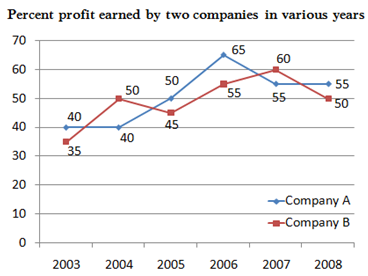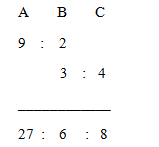# “20-20” Quantitative Aptitude | Crack SBI Clerk / IDBI Executive 2018 Day-60

Dear Friends, SBI Clerk 2018 Notification has been released we hope you all have started your preparation. Here we have started New Series of Practice Materials specially for SBI Clerk / IDBI Executive 2018 . Aspirants those who are preparing for the exams can use this “20-20” Quantitative Aptitude Questions.

[WpProQuiz 1770]

Click “Start Quiz” to attend these Questions and view Solutions

Click Here for SBI Clerk Prelims and Mains 2018 – Full Length Mock Test

Directions (Q. 1 – 5): What should come in place of question mark (?) in the following number series?

1. 256, 250, 230, 188, 116,?
1. 12
2. 6
3. 18
4. 9
5. None of these
1. 37, 39, 51, 87,?
1. 98
2. 124
3. 135
4. 167
5. None of these
1. 21, 28, 38, 52, 71, ?
1. 112
2. 84
3. 96
4. 138
5. None of these
1. 27, 27, 54, 18, 72, ?
1. 14.4
2. 19.6
3. 24.2
4. 29.8
5. None of these
1. 12, 13, 28, 87, ?
1. 264
2. 352
3. 116
4. 198
5. None of these

Directions (Q. 6 – 10): What should come in place of question mark (?) in the following questions?

1. 888 ÷ 12 + 2756 ÷ 13 + 1472 ÷ 16 = x % of 720
1. 48.5
2. 25.7
3. 52.5
4. 92.8
5. None of these
1. (5281 + 8604 + 1235) ÷ (491 + 769 + 252) =?
1. 15
2. 12
3. 18
4. 10
5. None of these
1. 152 – 342 + (8029 + 671) ÷ ? = 229
1. 7.5
2. 11.5
3. 15
4. 21
5. None of these
1. (3/18) of 2286 + (2/11) of 2431 =?
1. 652
2. 981
3. 716
4. 823
5. None of these
1. 92 × 152 ÷ √625 = 9x
1. 6
2. 3
3. 5
4. 7
5. None of these
1. A, B and C together have Rs. 16400. If 2/15th of A’s amount is equal to 3/5th of B’s amount and 3/4th of  B’s amount is equal to 9/16th of C’s amount, then how much amount does A have?
1. 10800
2. 7200
3. 8400
4. 9600
5. None of these
1. P, Q and R entered into a partnership by investing Rs. 60000, Rs. 70000 and Rs. 80000 respectively. After 5 months, P withdraws 30 % of the initial investment and Q invested 15000 more. And after 3 months, R withdraws 20 % of initial investment. Find the Q’s share, if total profit at the end of the year is Rs. 58440.
1. 18940
2. 15670
3. 25430
4. 22680
5. None of these
1. 5 years ago, the ages of Anu and Hema are in the ratio of 6 : 5. Three years ago, Anu’s age is equal to Hema’s age after 2 years. Then find the present age Anu?
1. 30 years
2. 35 years
3. 20 years
4. 25 years
5. None of these
1. A can do a piece of work in 20 days, B can do it in 25 days. They started the work together but A left 5 days before the completion of the work. The work has finished in?
1. 11 5/9 days
2. 15 6/7 days
3. 13 8/9 days
4. 18 1/7 days
5. None of these
1. The difference between length and breadth of the rectangle is 16. The length of the rectangle is equal to the side of the square, if the area of the square is 1024 Sq m. Find the perimeter of the rectangle?
1. 96 m
2. 88 m
3. 74 m
4. 112 m
5. None of these

Directions (Q. 16 – 20): Study the following graph carefully and answer the questions given below it.1. If income of company A in 2004 was equal to its expenditure in 2007, what was the respective ratio between the expenditure of company in 2004 and 2007?
1. 2 : 3
2. 5 : 7
3. 4 : 5
4. Can’t be determined
5. None of these
1. If income of company B in 2006 was Rs. 21.5 lakhs and ratio of income of company A and B was 4 : 5, what was approximate expenditure of company A in 2006?
1. Rs.16 Lakh
2. Rs.21 Lakh
3. Rs.8 Lakh
4. Rs.19 Lakh
5. Rs.10 Lakh
1. If total expenditure of both companies in 2008 was Rs. 15 lakhs and ratio of expenditure of company A and B in that year was 3 : 2 respectively what was income of company B in that year?
1. Rs.6 lakh
2. Rs.9 Lakh
3. Rs.12 Lakh
4. Rs.18 Lakh
5. None of these
1. If income of company A in 2003 was equal to expenditure of company B in 2004, what was the ratio of expenditure of company A in 2003 and income of company B in 2004?
1. 17 : 21
2. 10 : 23
3. 10 : 21
4. 7 : 13
5. None of these
1. If total income of company A in all the years together was equal to total expenditure of company B in all the years together which was Rs. 485 lakhs, what was profit percent earned by company A in all the years together?
1. 38
2. 40
3. 56
4. Can’t be determined
5. None of these

Directions (Q. 1 – 5):

The pattern is, -(32 – 3), -(52 – 5), -(72 – 7), -(92 – 9), -(112 – 11),..

(Or)

Third difference is 8, 8, 8

The pattern is, +(12 + 13), +(22 + 23), +(32 + 33), +(42 + 43),..

The difference of difference is, 3, 4, 5, 6,..

The pattern is, ÷ 1, *2, ÷ 3, *4, ÷ 5,..

The pattern is, *1 + 1, *2 + 2, *3 + 3, *4 + 4….

Directions (Q. 6 – 10):

(888/12) + (2756/13) + (1472/16) = (x/100)*720

74 + 212 + 92 = (x/100)*720

378 = (x/100)*720

X = (378*100) /720

X = 52.5

15120 / 1512 = x

X = 10

225 – 1156 + (8700/x) = 229

(8700/x) = 229 – 225 + 1156

(8700/x) = 1160

X = 8700/1160

X = 7.5

(3/18) * 2286 + (2/11) * 2431 = x

X = 381 + 442

X = 823

92 × (15 * 15)/ 25 = 9x

9x = 9 × 92

9x = 93

X = 3

A + B + C = Rs. 16400

(2/15)* A = (3/5)* B

(A/B) = (3/5) * (15/2) = 9/2

(3/4)* B = (9/16)* C

(B/C) = (9/16) * (4/3) = ¾41’s = 16400

1’s = 400

27’s = 10800

The ratio of share of P, Q and R

= > [60000*5 + 60000*(70/100)*7]: [70000*5 +85000*7] : [80000*8 + 80000*(80/100)*4]

= > 594000 : 945000 : 896000

= > 594 : 945 : 896

2435’s = Rs. 58440

1’s = 24

Q’s share = 945*24 = Rs. 22680

5 years ago, Anu : Hema = 6 : 5(6x , 5x)

Present ages of Anu and Hema = 6x + 5, 5x + 5

Three years ago, Anu’s age is equal to Hema’s age after 2 years,

= > 6x +2 = 5x + 7

= > x = 5

Present age of Anu = 6x + 5 = 35 years

(x – 5)/20 + x/25 = 1

(5x – 25 + 4x)/100 = 1

9x – 25 = 100

9x = 125

X = 125/9 = 13 8/9 days

Length = Side of square

Area of square = 1024 = a2

Side (a) = 32 m = length

According to the question,

32 – b = 16

Breadth = 32-16 = 16 m

Perimeter of the rectangle = 2(l + b) = 2(32 + 16)

= > 2(48)

= > 96 m

Directions (Q. 16 – 20):

Let the Income of Company A in 2004 and expenditure of company A in 2007 be x,

Expenditure of Company A in 2004

= > x = exp*(140/100)

= > exp = 100x/140

Required ratio = (100x/140) : x = 5 : 7

Income of company A in year 2006 = 4/5 x 21.5 = Rs. 17.2 lakh

If expenditure of company A in year 2006 is Rs. X

Profit % = {(I – E)/E} *100

Then, 65 = [17.2-x/x]*100

=> x = 1720/165 = Rs. 10 Lakh (Approx.)

Expenditure of Company B = 2 x 15/5 = Rs. 6 lakh

If income of company B in year 2006 is Rs. x, then

50 = {(x-6)/6}*100

x = Rs. 9 lakh

Let the Income of Company A in 2003 and expenditure of company B in 2004 be x,

Expenditure of Company A in 2003

= > x = exp*(140/100)

= > exp = 100x/140

Income of Company B in 2004

= > Inc = x*(150/100)

Ratio = 100x /140 : 150x /100 = 10 : 21

We can’t find the answers by given data.

Daily Practice Test Schedule | Good Luck

 Topic Daily Publishing Time Daily News Papers & Editorials 8.00 AM Current Affairs Quiz 9.00 AM Logical Reasoning 10.00 AM Quantitative Aptitude “20-20” 11.00 AM Vocabulary (Based on The Hindu) 12.00 PM Static GK Quiz 1.00 PM English Language “20-20” 2.00 PM Banking Awareness Quiz 3.00 PM Reasoning Puzzles & Seating 4.00 PM Daily Current Affairs Updates 5.00 PM Data Interpretation / Application Sums (Topic Wise) 6.00 PM Reasoning Ability “20-20” 7.00 PM English Language (New Pattern Questions) 8.00 PM General / Financial Awareness Quiz 9.00 PM